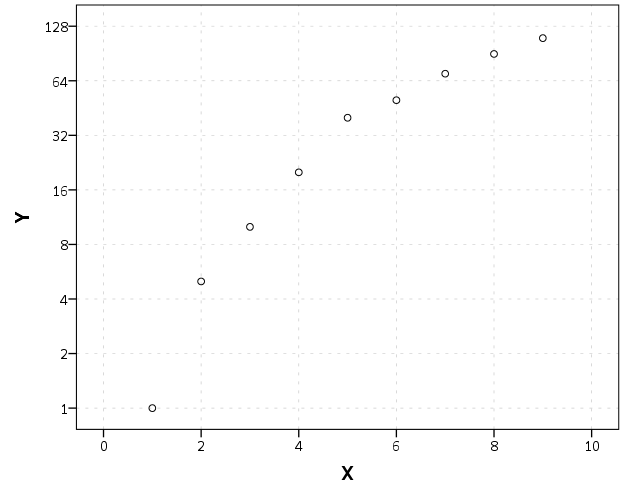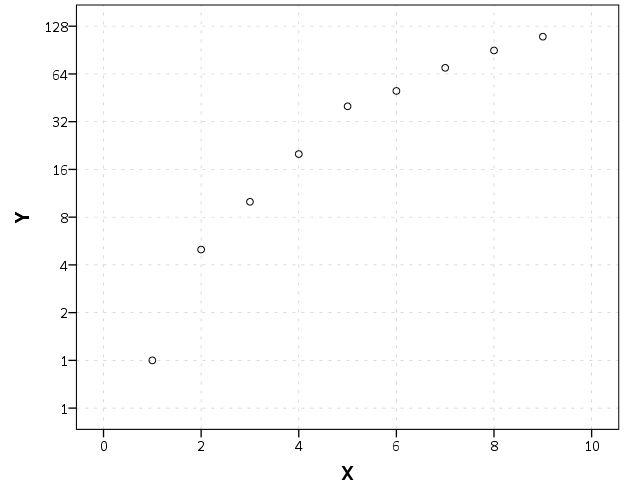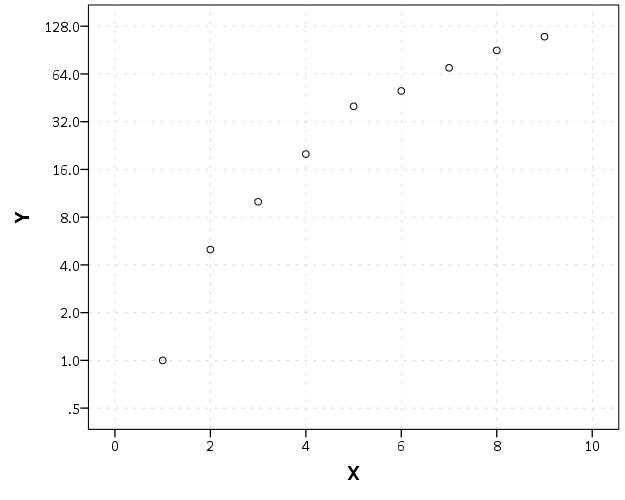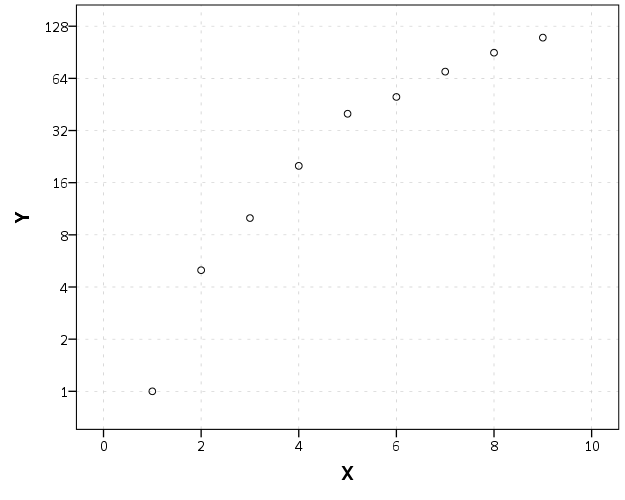# Log Scaled Charts in SPSS

Log scales are convenient for a variety of data. Here I am going to post a brief tutorial about making and formatting log scales in SPSS charts. So first lets start with a simple set of data:

``````DATA LIST FREE / X (F1.0) Y (F3.0).
BEGIN DATA
1 1
2 5
3 10
4 20
5 40
6 50
7 70
8 90
9 110.
END DATA.
DATASET NAME LogScales.
VARIABLE LEVEL X Y (SCALE).
EXECUTE.``````

In GPL code a scatterplot with linear scales would simply be:

``````GGRAPH
/GRAPHDATASET NAME="graphdataset" VARIABLES=X Y
/GRAPHSPEC SOURCE=INLINE.
BEGIN GPL
SOURCE: s=userSource(id("graphdataset"))
DATA: X=col(source(s), name("X"))
DATA: Y=col(source(s), name("Y"))
GUIDE: axis(dim(1), label("X"))
GUIDE: axis(dim(2), label("Y"))
ELEMENT: point(position(X*Y))
END GPL.``````

To change this chart to a log scale you need to add a `SCALE` statement. Here I will specify the Y axis (the 2nd dimension) as having a logarithmic scale by inserting `SCALE: log(dim(2), base(2))` between the last `GUIDE` statement and the first `ELEMENT` statement. When using log scales, many people default to having a base of 10, but this uses a base of 2, which works much better with the range of data in this example. (Log base 2 also works much better for ratios that don’t span into the 1,000’s as well.)

``````GGRAPH
/GRAPHDATASET NAME="graphdataset" VARIABLES=X Y
/GRAPHSPEC SOURCE=INLINE.
BEGIN GPL
SOURCE: s=userSource(id("graphdataset"))
DATA: X=col(source(s), name("X"))
DATA: Y=col(source(s), name("Y"))
GUIDE: axis(dim(1), label("X"))
GUIDE: axis(dim(2), label("Y"))
SCALE: log(dim(2), base(2))
ELEMENT: point(position(X*Y))
END GPL.``````Although the order of the commands makes no difference, I like to have the `ELEMENT` statements last, and then the prior statements before and together with like statements. If you want to have more control over the scale, you can specify and `min` or a `max` for the chart (by default SPSS tries to choose nice values based on the data). With log scales the minimum needs to be above 0. Here I use `0.5`, which is an equivalent distance from 1 -> 2 on a log scale.

``````GGRAPH
/GRAPHDATASET NAME="graphdataset" VARIABLES=X Y
/GRAPHSPEC SOURCE=INLINE.
BEGIN GPL
SOURCE: s=userSource(id("graphdataset"))
DATA: X=col(source(s), name("X"))
DATA: Y=col(source(s), name("Y"))
GUIDE: axis(dim(1), label("X"))
GUIDE: axis(dim(2), label("Y"))
SCALE: log(dim(2), base(2), min(0.5))
ELEMENT: point(position(X*Y))
END GPL.``````You can see here though we have a problem — two 1’s in the Y axis! SPSS inherits the formats for the axis from the data. Since the Y data are formatted as F3.0, the 0.5 tick mark is rounded up to 1. You can fix this by formatting the variable before the `GGRAPH` command.

``````FORMATS Y (F4.1).
GGRAPH
/GRAPHDATASET NAME="graphdataset" VARIABLES=X Y
/GRAPHSPEC SOURCE=INLINE.
BEGIN GPL
SOURCE: s=userSource(id("graphdataset"))
DATA: X=col(source(s), name("X"))
DATA: Y=col(source(s), name("Y"))
GUIDE: axis(dim(1), label("X"))
GUIDE: axis(dim(2), label("Y"))
SCALE: log(dim(2), base(2), min(0.5))
ELEMENT: point(position(X*Y))
END GPL.``````But this is annoying, as it adds a decimal to all of the tick values. I wish you could use fractions in the ticks, but this is not possible that I know of. To prevent this you should be able to specify the `start()` option in the `GUIDE` command for the second dimension, but in a few attempts it was not working for me (and it wasn’t a conflict with my template — in this example set the `DEFAULTTEMPLATE=NO` to check). So here I adjusted the minimum to be `0.8` instead of `0.5` and SPSS did not draw any ticks below 1 (you may have to experiment with this based on how much padding the Y axis has in your default template).

``````FORMATS Y (F3.0).
GGRAPH
/GRAPHDATASET NAME="graphdataset" VARIABLES=X Y
/GRAPHSPEC SOURCE=INLINE DEFAULTTEMPLATE=YES.
BEGIN GPL
SOURCE: s=userSource(id("graphdataset"))
DATA: X=col(source(s), name("X"))
DATA: Y=col(source(s), name("Y"))
GUIDE: axis(dim(1), label("X"))
GUIDE: axis(dim(2), label("Y"), start(1))
SCALE: log(dim(2), base(2), min(0.8))
ELEMENT: point(position(X*Y))
END GPL.``````I will leave talking about using log scales if you have 0’s in your data for another day (and talk about displaying missing data in the plot as well.) SPSS has a `safeLog` scale, which is good for data that has negative values, but not necessarily my preferred approach if you have count data with 0’s.

Next Post

1.#### Bartje

/  August 1, 2020

Thanks a lot!
Curious about how to deal with zero’s though! Any solution on this?

•